Higher-Order ODEs: the Beam Equation

Set up the ODE solution (we will specify a unit beam stiffness) EI = 1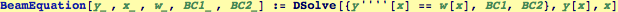Functions for Typical Boundary Conditions and Beam Loading

Set up some typical boundary conditions:

A clamp fixes the position and the angle of a beam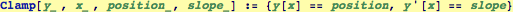The position of a beam and the applied moment (or torque) is applied with a Knob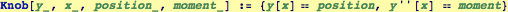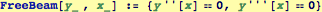Set up some typical beam loads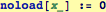A unit load is a force of 1/length applied over the beam length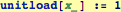midload appliec a fixed load at x=1/2.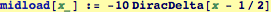Boxload simulaties a "box" of a fixed width and weight per unit width placed at x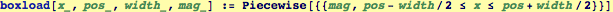Simulated linearly decreasing distributed load (pushes up when x<1/2; down for x > 1/2)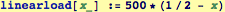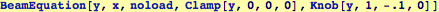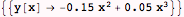Created by Wolfram Mathematica 6.0  (16 November 2007)